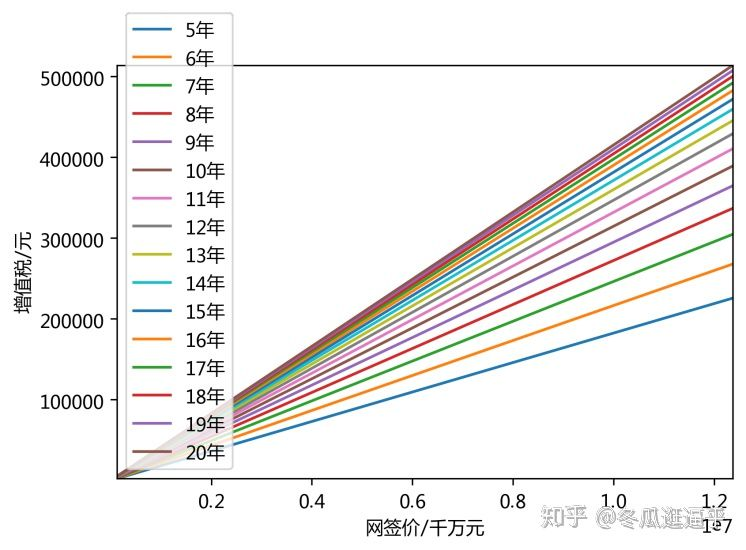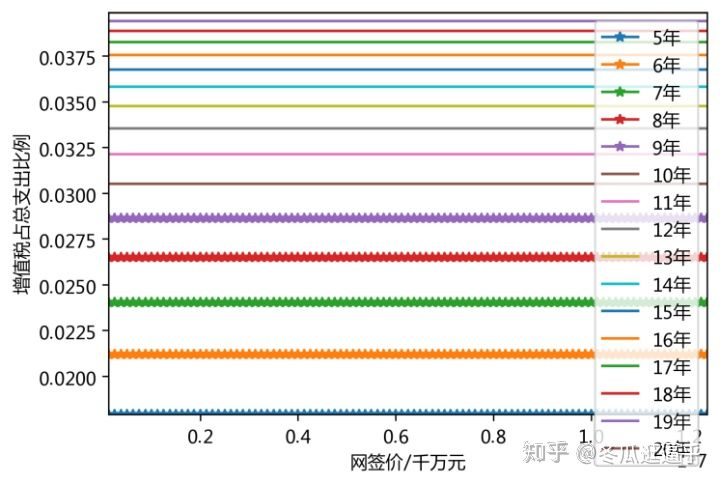# 增值税及其附加税

< 2 年 (税务核定价 ÷ 1.05) × (5% + 0.55%)
[2, 5) 年 免征
≥ 5 年 免征

< 2 年 (税务核定价 ÷ 1.05) × (5% + 0.55%)
[2, 5) 年 ((税务核定价- 买入价格) ÷ 1.05) × (5% + 0.55%)
≥ 5 年 ((税务核定价 - 买入价格) ÷ 1.05) × (5% + 0.55%)

## 核定价影响贷款额度

$\begin{equation} \left\{ \begin{array}{lr} T_{贷款额度} = T_{核定} \times 65\% \notag \\ T_{核定} = T_{网签} \times 85\% \notag \\ \end{array} \\ \right. \end{equation}$

$\begin{equation} \left\{ \begin{array}{lr} T_{贷款额度} = T_{网签} \times 55.25\% \notag \\ T_{首付} = 1-55.25\% = 44.75\% \notag \\ \end{array} \right. \end{equation}$

# 增值税影响下的购房决策分析

\begin{align} T_{差额} &= T_{核定价} - T_{买入价格} \notag \\ \ &= 0.85T_{网签} - T_{网签} \div (1 + 0.1463)^y \notag \\ \end{align}

\begin{equation} T_{增值税} = \left\{ \begin{aligned} & \frac{T_{核定价}}{1.05} \times 5.55\% = 0.04497 · T_{网签} && (y < 2)\\ & 0 && (y \geq 2) \end{aligned} \\ \right. \notag \\ \end{equation}

\begin{equation} T_{增值税} = \left\{ \begin{aligned} & 0.04497 · T_{网签} && (y < 2) \notag \\ \\ & (0.85T_{网签} - \frac{T_{网签}}{(1 + 0.1463)^y}) \times 0.0529 && (y \geq 2) \notag \\ \end{aligned} \right. \notag \\ \end{equation}

## 非普通住宅的年限影响1. 房龄越高，其所得税缴纳越多。这也是可以理解的，因为随着房价的上涨，其差额会逐渐变大。
2. 所得税的增长幅度与年限呈现下降趋势。随着年限增高，增长差值逐渐降低。

## 增值税占比

$P_{增值税所占比例} \approx \frac{T_{增值税}}{T_{网签价} + T_{增值税}}$# 总结

1. 购买二手房时，首付高一些，将近 50%。 其原因是因为税务核算价往往偏低，从而拉低了贷款比重，进而使得首付提高；
2. 当购买非普通住宅时，房龄越高，其所得税缴纳越多。因为随着房价的上涨，其差额会逐渐变大。
3. 所得税的增长幅度与年限呈现下降趋势。随着年限增高，增长差值逐渐降低。
4. 确定需求面积所支出的总价，尽可能的去购买新房。这与我们的正常认知也是相同的。

# 相关数据分析代码

"""

"""
# encoding=utf-8
import numpy as np
import math
from matplotlib import pyplot
import matplotlib.pyplot as plt
from IPython.core.pylabtools import figsize # import figsize
#figsize(12.5, 4) # 设置 figsize
plt.rcParams['savefig.dpi'] = 300 #图片像素
plt.rcParams['figure.dpi'] = 300 #分辨率

# 总价
ths = [i * 125000 for i in range(1, 100)]

# 年限
ys = [i for i in range(5, 21)]

for y in ys:
# 增值税计算
trs = []
for th in ths:
tr = (0.85 * th - th / ((1 + 0.1463) ** y)) * 0.0529
trs.append(tr)

plt.plot(ths, trs, label=f'{y}年')

plt.legend()
plt.margins(0)
plt.xlabel('网签价/千万元') #X轴标签
plt.ylabel("增值税/元") #Y轴标签

"""

"""
# 年限
ys = [i for i in range(5, 21)]

for y in ys:
# 增值税计算
trs = []
for th in ths:
tr = (0.85 * th - th / ((1 + 0.1463) ** y)) * 0.0529
trs.append(tr / (th + tr))
print(sum(trs) / len(trs))
if y >= 10:
plt.plot(ths, trs, label=f'{y}年')
else:
plt.plot(ths, trs, marker='*', label=f'{y}年')

plt.legend()
plt.margins(0)
plt.xlabel('网签价/千万元') #X轴标签
plt.ylabel("所得税占总支出比例") #Y轴标签

"""

"""
p = [
0.017910502320842965,
0.021189641628359904,
0.024032438906425032,
0.026498963556469416,
0.028640531033586977,
0.030501094013867885,
0.032118385667131544,
0.03352486519307519,
0.034748504347376426,
0.035813445129716175,
0.03674055234381547,
0.03754787981470654,
0.038251065262737784,
0.03886366589974932,
0.03939744452077101,
0.039862614060287876,
]

plt.plot(p, y, marker='o')
plt.legend()
plt.margins(0)
plt.xlabel('增值税占总支出比例') #X轴标签
plt.ylabel("房子年限/年") #Y轴标签